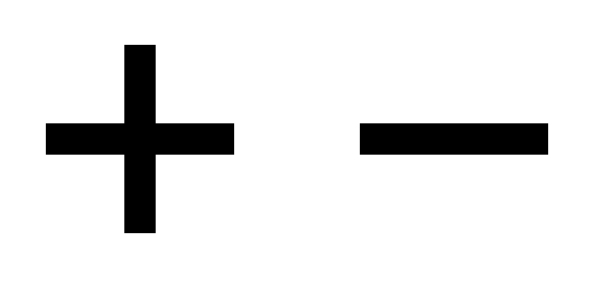# 2 And 3-digits Addition And Subtraction

Approved & Edited by ProProfs Editorial Team
The editorial team at ProProfs Quizzes consists of a select group of subject experts, trivia writers, and quiz masters who have authored over 10,000 quizzes taken by more than 100 million users. This team includes our in-house seasoned quiz moderators and subject matter experts. Our editorial experts, spread across the world, are rigorously trained using our comprehensive guidelines to ensure that you receive the highest quality quizzes.
| By Henrisat
H
Henrisat
Community Contributor
Quizzes Created: 1 | Total Attempts: 574
Questions: 5 | Attempts: 574Settings• 1.

### Add. Regroup if you need to.  347+58

• A.

405

• B.

459

• C.

345

• D.

289

A. 405
Explanation
To find the sum of 347 and 58, we can add the numbers digit by digit. Starting from the rightmost digit, 7 plus 8 equals 15. Write down the 5 and carry over the 1. Moving to the next digit, 4 plus 5 plus the carried over 1 equals 10. Write down the 0 and carry over the 1. Finally, add the carried over 1 to the leftmost digit 3. The sum is 405.

Rate this question:

• 2.

### Subtract. Regroup if you need to.  53-47

6
Explanation
When subtracting 47 from 53, we start by subtracting the ones place, which gives us 3. Then, we subtract the tens place, which gives us 1. Therefore, the difference between 53 and 47 is 6.

Rate this question:

• 3.

### 478 > 509

• A.

True

• B.

False

B. False
Explanation
The given statement "478 > 509" is false. In numerical order, 509 is greater than 478. Therefore, the correct answer is False.

Rate this question:

• 4.

### Sarah is working in the garden. She planted  23 daisies , 15 roses and 40 sunflowers.How many flowers did Sarah planted in all ?

• A.

67

• B.

103

• C.

78

• D.

88

C. 78
Explanation
Sarah planted 23 daisies, 15 roses, and 40 sunflowers. To find the total number of flowers she planted, we add these quantities together: 23 + 15 + 40 = 78. Therefore, Sarah planted a total of 78 flowers.

Rate this question:

• 5.

### Georges went to the store with 5.00 in his pocket. He bought  two lolipop for 1.00 each. then he got a candy for 50 cents. Finally he bougth a yo-yo for \$1.50. How much money he has left?

• A.

\$ 4.00

• B.

\$ 1.50

• C.

\$ 1.00

• D.

\$ 0.00

C. \$ 1.00
Explanation
Georges started with \$5.00 and spent \$1.00 on two lollipops, \$0.50 on a candy, and \$1.50 on a yo-yo. Therefore, he spent a total of \$3.00. To find out how much money he has left, we subtract the amount spent from the initial amount. \$5.00 - \$3.00 = \$2.00. Therefore, he has \$2.00 left. However, none of the given answer choices match this amount, so the correct answer is not available.

Rate this question:

Related TopicsBack to top